# Maximum Flow Assumptions

 Maximum Flow Notation Maximum Flow Assumptions 1. Network is directed 2. All capacities are non-negative integers 3. Network does not have a path from s to t with all arcs having infinite capacity 4. If (i, j)  A then (j, i)  A  Nonrestrictive because we allow arcs with capacity = 0 5. Network has no parallel arcs Applications Feasible flow problem (application 6.1)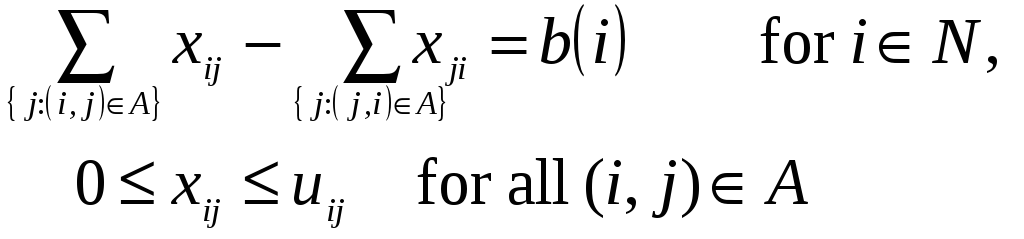As before, we assume that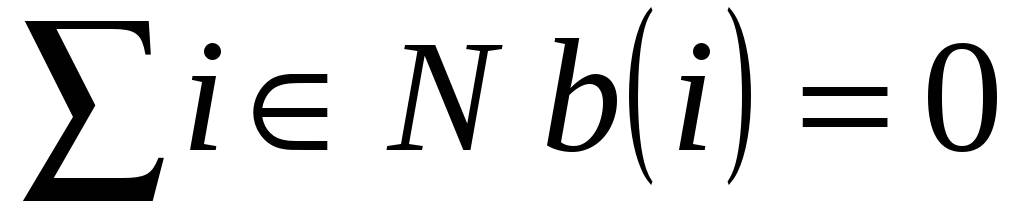Introduce two new nodes A source node s and a sink node t. For each node i with b(i)>0, we add an arc (s, i) with capacity b(i). For each node i with b(i)<0, we add an arc (i, t) with capacity -b(i). We refer to the new network as the transformed network. Then we solve a maximum flow problem from node s to node t in the transformed network. If the maximum flow saturates all the source and sink arcs, problem (6.2) has a feasible solution; otherwise, it is infeasible. Additional Applications 1. Pipeline 1 3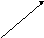s t 2 4 Definitions and Notation Residual Network Given a flow x, the residual capacity, rij, of an arc (i, j)A is the maximum additional flow that can be put on (i, j). Note that there will also be an rji [recall assumption] rij has two components (1) uij - xij unused capacity of (i, j) (2) xji flow on (j, i) which we can cancel to increase the flow from i to j rij = uij - xij + xji xij, uij Example i j 2 1 4 3 rij Residual network i j 2 1 4 3 s - t cut Cut partition N into two sets, S and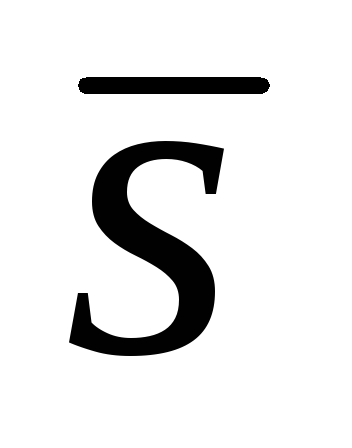= N - S Notation: [S,] or arcs with one endpoint in S and one ins-t cut if s  S & t Forward arc of cut if (i, j) i  S j set denoted (S,) Backward arc of cut if (i, j) i j  S set denoted (, S) Example 2 4s t 3 (S,) = (2, 4), (3, 4), (3, t) (, S) =  Capacity of an s - t cut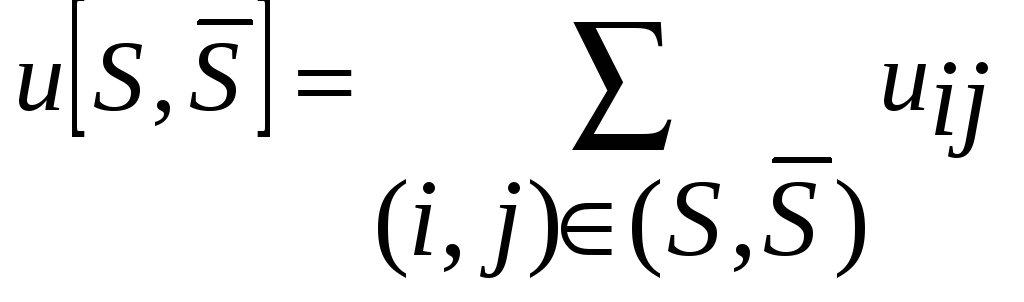Minimum cut Residual capacity of s - t cut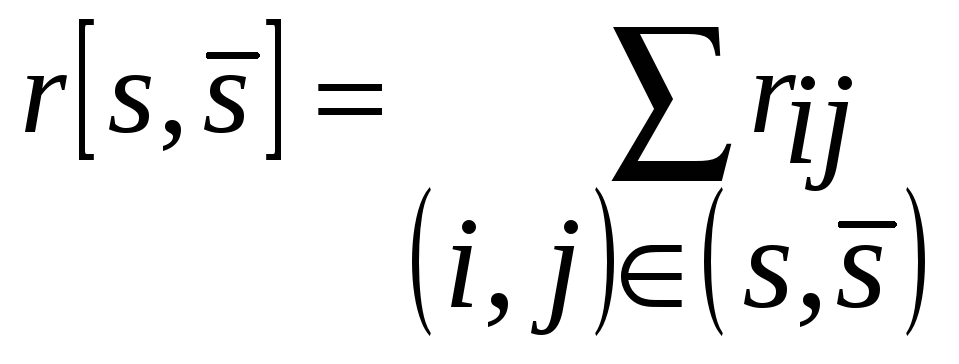Download 1 Mb.Share with your friends: